# MCAT Physical : Force Equilibrium

## Example Questions

← Previous 1

### Example Question #1 : Force Equilibrium

Which of the following scenarios describes a system that is not in equilibrium?

A skydiver that has deployed his parachute and is falling straight down at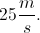A racecar driver driving at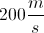around a mile-long circular track

A tightrope walker balancing on one foot on a wire

All of these scenarios describe systems in equilibrium

A racecar driver driving ataround a mile-long circular track

Explanation:

The best way to think of a system in equilibrium is that it is displaying a constant velocity in both the horizontal and vertical directions. Both the skydiver and the tightrope walker have a constant velocity. The driver, however, is driving around a circular track. You must remember that velocity is a vector of magnitude and direction. Because the direction of the driver is changing, he is not driving at a constant velocity; thus, he is not in equilibrium, and is experiencing an acceleration (centripetal acceleration).

Keep in mind that the skydiver is experiencing both the downward force of gravity, and the upward force of air resistence on his deployed parachute. These forces cancel, producing a net force of zero, and allowing him to fall at a constant velocity in equilibrium.

### Example Question #1 : Force Equilibrium

Three forces are acting on an object. One force acts on the object to the north, and another force acts on it to the west. The object is accelerating directly westward when all three forces are acting on it.

Based on this information, which of the following statements must be true?

The third force is stronger than the western force

The third force is weaker than the western force

The third force is stronger than the northern force

The third force must have some force in the southern direction

The third force must have some force in the southern direction

Explanation:

Since the only acceleration on the object is to the west, we can conclude that there is no vertical acceleration on the object. As a result, the northern force must be offset by the third force. The only way to offset the northern force is to have a force pointing south, in any direction. As such, the third force could be directly south, southwest, or southeast as long as the southern component can counteract the northern force.

The third force would only need to be equal to the northern force if it was pointing directly due south, and we can only confirm that the third force is not completely offsetting the western force. As a result, we can only conclude that the third force has some magnitude in the southern direction.

### Example Question #3 : Force Equilibrium

As a skydiver jumps out of a plane, he comes to terminal velocity as air resistance brings his fall to a constant speed. At what point is the net force on the skydiver the greatest?

The net force is constant through the fall

Right when he jumps out of the plane

When he has reached terminal velocity

When he is halfway to the earth's surface

Right when he jumps out of the plane

Explanation:

The skydiver’s velocity (along the y-axis) will be zero when he initially jumps out of the plane, however, his net force will be greatest at that point.

As the skydiver first jumps, there is only the force of gravity acting on him. As he approaches terminal velocity, however, the force of wind resistance becomes equal and opposite to the force of gravity, and he begins to move at constant velocity with a net force of zero.

### Example Question #2 : Force Equilibrium

A mass hanging in equilibrium is attached to a fixed surface on the ceiling by a spring. The mass is pulled down from the ceiling, then released and allowed to move in simple harmonic motion. The mass does not lose energy due to friction or air resistance. At what point does the mass have maximum velocity?

At maximum downward displacement from the equilibrium position

At the equilibrium position

At maximum upward displacement from the equilibrium position

Its velocity is constant

At the equilibrium position

Explanation:

The velocity of the mass will be the greatest at the equilibrium position. Although the restoring force on the mass due to the spring will be the greatest at maximum displacement from the equilibrium position, this force is being applied to the mass as it moves in the opposite direction. As the mass moves towards the equilibrium position from maximum displacement, the restoring force due to the spring is applied in the same direction as the velocity of the mass. Because of this, the object is accelerating. After the mass passes through the equilibrium position, the spring begins to apply a force in the direction opposite to the motion of the mass, resulting in the deceleration of the mass. The mass experiences its maximum velocity at the equilibrium position.

### Example Question #2 : Force Equilibrium

If a wooden block is decelerating across a surface, in what direction is the net force on the object?

The same direction as the motion

There is no net force

Initially in the direction of the motion, then opposite the direction of motion

Initially opposite the direction of motion, then in the direction of the motion

Opposite to the motion

Opposite to the motion

Explanation:

When an object is decelerating, there is a net force in the opposite direction of the motion of the object. Usually, this force is friction, which always acts counter to the net velocity. Even if there is a force in the direction of the object's motion, the net force is backwards if the object is decelerating.

Mathematically, we can prove this concept using Newton's second law. The direction of the object's motion will be positive, while the opposite (backwards) direction will be negative.

If the object is decelerating, then the acceleration is negative.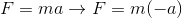From this calculation, the force must be negative, acting counter to the direction of motion.

### Example Question #221 : Newtonian Mechanics And Motion

What is the net force on a falling object at terminal velocity?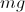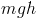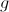Explanation:

When an object is at terminal velocity, the upward force on the object from air resistance is equal to the force of gravity. These equal, but opposing, forces cancel each other out and the net force is zero. This results in a zero acceleration, allowing the object to fall at a constant (terminal) velocity.

### Example Question #3 : Force Equilibrium

A 2kg mass is suspended on a rope that wraps around a frictionless pulley attached to the ceiling with a mass of 0.01kg and a radius of 0.25m. The other end of the rope is attached to a massless suspended platform, upon which 0.5kg weights may be placed. While the system is initially at equilibrium, the rope is later cut above the weight, and the platform subsequently raised by pulling on the rope.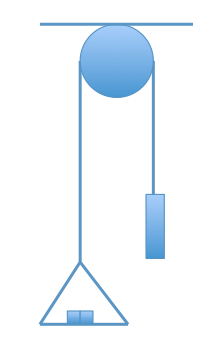What is the tension in the rope if the system is at equilibrium?

9.8N

23N

5N

19.6N

19.6N

Explanation:

Consider that tension is a contact force that acts over a distance. Assuming the rope does not stretch and has no mass (as we should for the MCAT), we can think of tension as a force that acts directly on the mass. We can draw a force diagram below.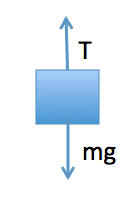If the system is stationary (at equilibrium), we can see that tension is equal to the weight, T = mg.

T = (2 kg)(9.8 m/s2) = 19.6N

### Example Question #8 : Force Equilibrium

Two positively charged particles are placed near one another in an otherwise isolated environment. Their charges are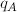and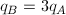, and their masses are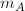and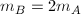. The force of gravity between these particles is negligible compared to the elecrostatic force which repels them.

At a particular moment, the magnitude of particle A's acceleration is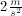. At this moment, what is the magnitude of particle B's acceleration?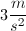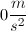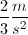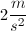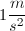Explanation:

Use Newton's second law,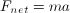, to relate the forces and accelerations of the particles. Writing this out for the two charges gives the following equations.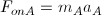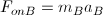The two charges exert equal-magnitude and opposite-direction forces on one another according to Newton's third law, so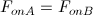, or using the right side of the second law equations,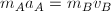.

Plugging in our given values allows us to solve for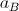.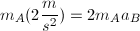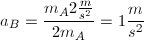### Example Question #9 : Force Equilibrium

A potted plant is hanging from a rope attached to a hook. The plant exerts 25N of force in the downward direction. Assume the rope is weightless.

What is the tension of the rope?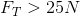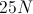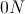The rope is weightless, so it cannot have tensionExplanation:

In order for the potted plant to stay still, the net force on the plant must be 0N. The tension of the rope is the force that allows an object to hang without falling. In order to keep the plant stationary, the tension must be equal to 25N in the upward direction.

### Example Question #14 : Forces

A skydiver with a mass of 100kg jumps out of an airplane and reaches a terminal velocity of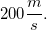.

What is the total force acting on the skydiver at this point in his jump?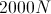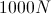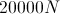The man has reached a terminal velocity, which means that he has an acceleration of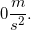Because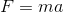, the total force on the skydiver is 0N. At this point, the force due to gravity in the downward direction is equal to the force of air resistance in the upward direction.